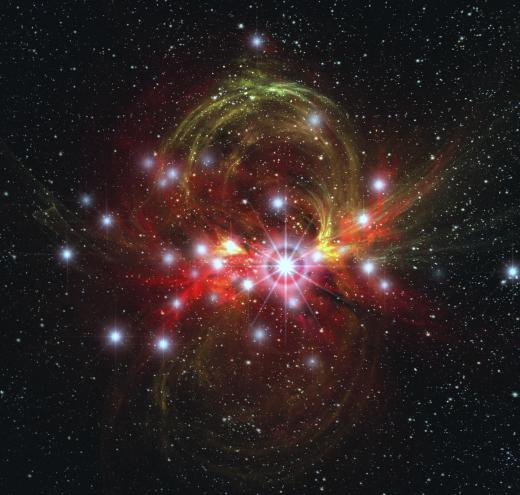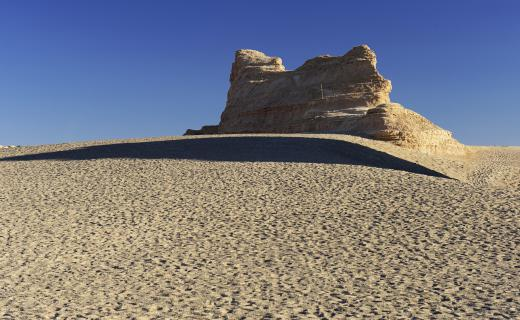Internet
Fact Checked

# What Is the Connection between Computer Simulation and Modelling?

Marco Sumayao
Marco Sumayao

Computer simulation and modelling almost always go hand-in-hand with each other, as computers use different types of models to carry out simulations. The two most common types of models used in computer simulation are mathematical models and three-dimensional models. A computer simulation can use one or both types of models to recreate abstract situations, the results of which will be an integral part of research and development. The simulation software will carry out different manipulations of the computer models to determine the effects of these actions. The applications of computer simulation and modelling are present in nearly every major scientific field, including biology, chemistry, and physics.

The dynamics of computer simulation and modelling are simple — developers create simulations according to their needs and design models for use in the simulations. A simulation is a computer program written to create certain situations and conditions, while a model is an object used within the simulation. Researchers often rely on computer simulation and modelling in order to study conditions that might otherwise be improbable — even impossible — to physically observe. A physicist, for example, might rely on a simulation to determine the effects of a supernova on nearby planets. Developers and marketers, on the other hand, might need a simulation to demonstrate the capabilities of a product not physically present.Researchers often use simulations to study objects that are not physically present, such as a supernova.

The models often represent manipulated objects in the simulation; these objects are subjected to different actions, with the effects recorded for review and presentation. A researcher can, for example, create a simulation for determining the effects of wind erosion on a rock. He will then create a computational model for a rock, with mathematical representations of its density, shape, and other characteristics. When the simulation is run, the researcher will subject the rock model to a wind program designed to virtually erode the stone.Geologists use simulations to determine how wind erosion created the crests and ridges of a yardang.

Models can also be created for static objects in the simulation — objects that are not manipulated by the program in any way. These are often used to define environmental constants and limitations. An individual running a simulation for a laser, for instance, could create a model for airborne particles that might be in the laser's path and potentially affect the simulation's end result. In cases where computer simulation and modelling are used primarily for visual presentations, the static models could represent the location in which other models move, or serve as objects that cannot be moved.

## You might also Like